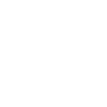### TA的资料

• 98回答
• 1提问
• 81%采纳率
• 6498帮助人数
• 262
• 67已被推荐、被采纳的回答数

### TA的勋章

•一路有你

•初学乍练

### 收到礼物

•顶牛仁

6

•送香吻

2

•鲜花雨

2

### TA的回答

• （8+8+8+ 8÷8）×[8+(8+8)÷8]×8+8=20088×[8×8×8-8-(8+8)÷8]÷(8+8)×8=2008

4  |  2015-05-03

推荐答案
• 前面对，后面不对2.8平方米 = 280平方分米；（1平方米=100平方分米）8立方米 = 8000立方分米；（1立方米=1000立方分米）

1  |  2015-05-03

推荐答案

1  |  2015-05-03

满意答案
• 因为 x^2 - 3x + 1 =0 （^是数的平方），则 x ≠ 0，且x^2 + 1 = 3x ,即（x^2 + 1）/x = 3 ,所以 ，  x^2 + 3/x + 1 = 3x+3/x=3(x^2 + 1)/x=9

1  |  2015-05-03

推荐答案
• 因为 (x-1)^2+(5-x)^2=15，即  x^2 -2x + 1 +（ x^2 -10x + 25）=15，2 x^2 -12x + 10= - 1，得 x^2 -6x + 5 = - 1/2，所以，  (x-1)^2×(5-x)^2 =[ (x-1)×(x-5)]^2=(x^2 -6x + 5)^2=(- 1/2)^2=1/4

1  |  2015-05-03

满意答案